### Home > MC1 > Chapter 6 > Lesson 6.2.2 > Problem6-58

6-58.
1. .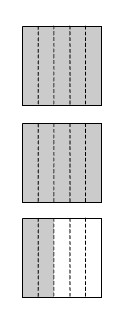George drew the diagram at right to represent the number 2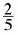. "Look," said Helena, "This is the same thing as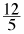." Homework Help ✎

1. Is Helena correct? If so, explain how she can tell that the diagram represents. If she is not correct, explain why not.

2. Draw a diagram to represent the mixed number 3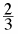. How can you write this as a single fraction greater than one?

3. How can you write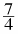as a mixed number? Be sure to include a diagram in your answer.

How much does each piece represent?

Since each square is divided into 5 equal pieces, each piece is equal to one-fifth.
There are 12 shaded pieces, so this diagram represents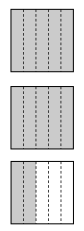$\frac{12}{5}.$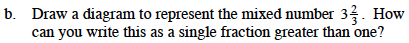Use the diagram for part (a) to help you design this diagram.
How many pieces are in a whole? How many do you shade?

To represent this mixed number as a single fraction greater than one, 11 equal pieces will need to be shaded.
How much of a whole will each piece represent?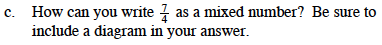How many whole numbers does this represent?
How many pieces are left over?
Remember to draw a diagram.